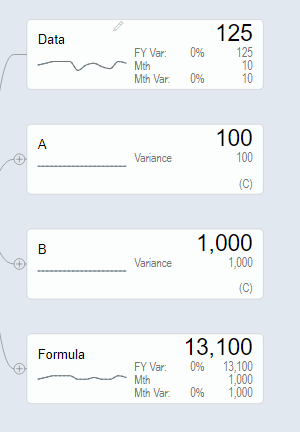# Formula using constant to recalculate data in model in sim

1.97K views
0

Hi, in my scenario I need as part of the simulation modify constant which is a component of the node formula whereas the formula should recalculate every row in the dataset and compare it in the MAX fn:

SUM (

MAX (

EVERY_DATASET_ROW * NODE_CONSTANT_A,

NODE_CONSTANT_B

)

)

Currently the result I am getting is SUM ( EVERY_DATASET_ROW * NODE_CONSTANT_A ), which is incorrect. Any help appreciated.

0

Hi,

We tried to recreate your query using the following steps.

1. Data node with row values for 12 periods, 2 constant nodes – A and B with values as 100 and 1000 respectively and a formula node2. Formula entered is as seen in the image3. Result  of the formula:
1. In valQ2. In ExcelThe formula seems to be working in the above example.